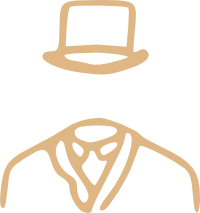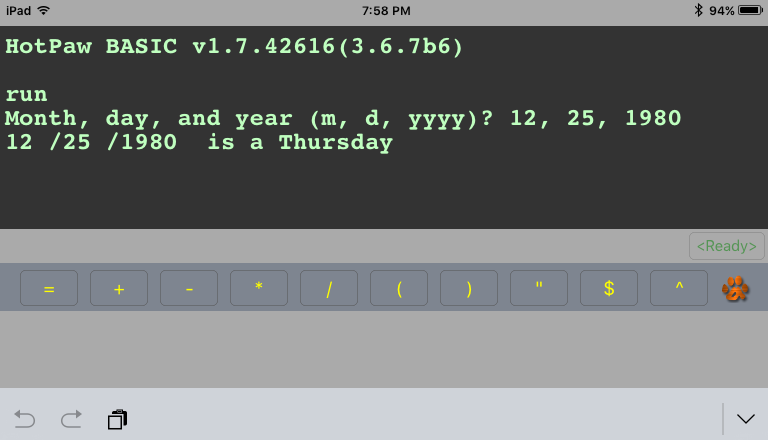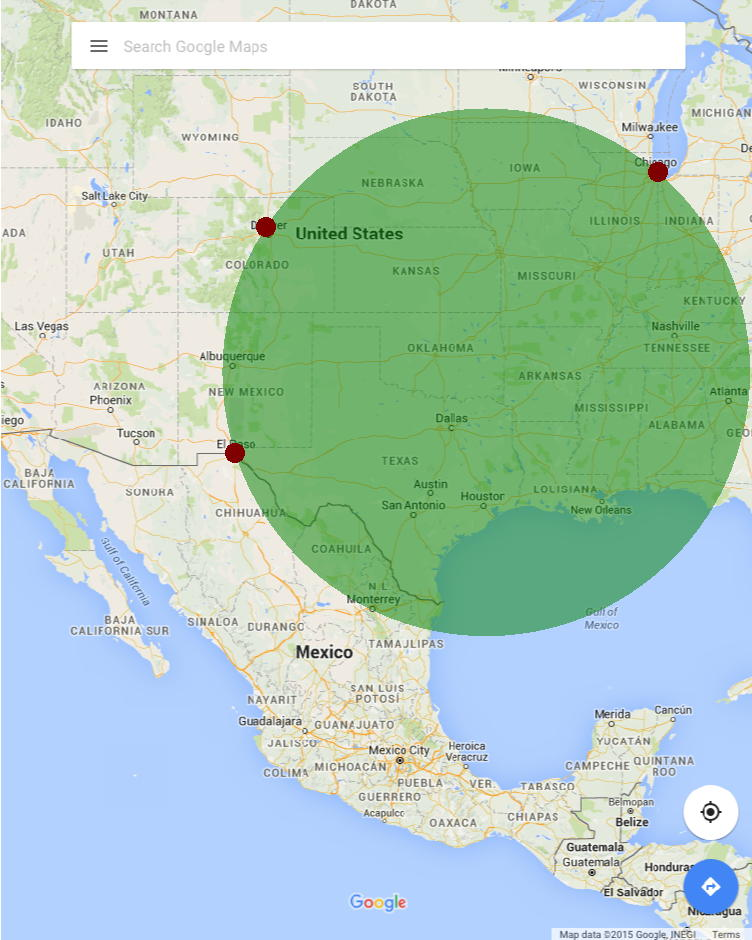# Mimsy Were the Borogoves

Hacks: Articles about programming in Python, Perl, Swift, BASIC, and whatever else I happen to feel like hacking at.

# Draw a circle on an iPad map from three points in Pythonista

I happened across a copy of 57 Practical Programs & Games in BASIC while traveling a month ago, and was once again fascinated by the simple toolchests we used back in the seventies and eighties. I fooled around with it a bit, typing up the programs in HotPaw BASIC on the iPad. I now have the BASIC code in HotPaw necessary to tell what day of the week any date, post 1752, fell on, as well as a Chi-Square evaluator that I’ll probably never use.While reading through it, I came across the code for Circle determined by three points and thought about how cool it would be to use that simple code on modern mobile tools. It should be a snap to write an on-the-fly app in HotPaw BASIC or Pythonista. Take a snapshot of a map, tap three points, and see what the circle is.

HotPaw BASIC does not appear to have access to the iPad’s photo library, but Pythonista does. It has a photos module that allows you to pick_image and several methods in the scene module for simple manipulations and display.

The code itself is pretty simple. Create a scene, override touch_end to capture points (touch_end is similar to onClick in JavaScript), and the BASIC code from 57 Programs converted to Python, to determine the center and radius of a circle given three points on the screen.

[toggle code]

• from scene import *
• import photos
• class MyScene(Scene):
• def __init__(self, mapimage):
• #scene.image can only display RGBA images
• self.mapimage = mapimage.convert('RGBA')
• self.points = []
• super(MyScene, self).__init__()
• def setup(self):
• # scale image to fit screen
• width = self.mapimage.size
• height = self.mapimage.size
• widthratio = width/self.size.w
• heightratio = height/self.size.h
• if widthratio &gt; heightratio:
• scale = widthratio
• else:
• scale = heightratio
• if scale != 1:
• width = int(width/scale)
• height = int(height/scale)
• self.mapimage = self.mapimage.resize((width, height))
• # center the image on the screen
• self.imagelocation = [(self.size.w-width)/2, (self.size.h-height)/2]
• # load image for display
• def draw(self):
• background(1, 1, 1)
• image(self.mapimage, *self.imagelocation)
• #if there are three points, draw a circle
• if len(self.points) == 3:
• self.makeCircle(self.points)
• #if there are any points, show them
• if self.points:
• self.showPoints(self.points)
• #override touch_ended to store/remove touches
• def touch_ended(self, touch):
• #if any of the touches are in a previous touch, remove them
• if self.remove_point(touch):
• return
• #if there are fewer than three touches, add this one
• if len(self.points) &lt; 3:
• self.points.append(touch)
• def remove_point(self, point):
• for existingPoint in self.points:
• #determine distance between tap and existing point
• x1 = existingPoint.location.x
• y1 = existingPoint.location.y
• x2 = point.location.x
• y2 = point.location.y
• distance = sqrt((x2-x1)**2 + (y2-y1)**2)
• #if the tap is in the circle of an existing point, delete it
• self.points.remove(existingPoint)
• return True
• return False
• def showPoints(self, points):
• fill(.5, 0, 0)
• for point in points:
• x = point.location.x
• y = point.location.y
• def makeCircle(self, points):
• #determine center and radius of circle from three points
• x1 = points.location.x
• x2 = points.location.x
• x3 = points.location.x
• y1 = points.location.y
• y2 = points.location.y
• y3 = points.location.y
• n1 = (y2-y1)/(x2-x1)
• n2 = (y3-y1)/(x3-x1)
• k1numerator = (x2-x1)*(x2+x1) + (y2-y1)*(y2+y1)
• k1 = k1numerator/(2*(x2-x1))
• k2numerator = (x3-x1)*(x3+x1) + (y3-y1)*(y3+y1)
• k2 = k2numerator/(2*(x3-x1))
• y = (k2-k1)/(n2-n1)
• x = k2-(n2*y)
• radius = sqrt((x3-x)**2 + (y3-y)**2)
• # ellipse draws in a rectangle, so x/y need to be the lower left corner
• fill(0, .5, 0, .5)
• mapimage = photos.pick_image(show_albums=True)
• if mapimage:
• scene = MyScene(mapimage)
• #the smaller the frame_interval, the more responsive it will be
• #and the faster the battery will drain
• run(scene, frame_interval=5)
• else:
• print 'Canceled or invalid image.'

This code asks the user to pick an image from any album. If you remove show_albums=True, it will only display images from the camera roll.

It then instantiates a MyScene instance given the chosen “mapimage”.

Inside, the current version of Pythonista as I write this requires that load_pil_image be in the setup method; future versions will allow it in the init method as well.1 So setup resizes the image either horizontally or vertically as necessary to fill the screen without distortion, then loads it for display using scene.image.

On every tap, the code checks to see if the user was tapping on an existing point; if so, that point is removed. Otherwise, as long as there are fewer than three points currently stored, it stores that point.

The draw method displays all of the points, and if there are three of them, displays the circle using the formula from 57 Programs.

Mostly worthless, but it seems like the kind of thing that might show up in a fraught race to find a criminal in a modern crime show. It should be possible to do a lot of cool programming on the fly on mobile devices using tools like Pythonista.A circle whose circumference lies on all three of Chicago, Denver, and El Paso.

1. Thanks to mmontague and JonB on the Pythonista forums for help tracking down where load_pil_image needed to be.